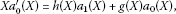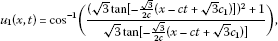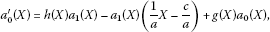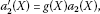We’d like to understand how you use our websites in order to improve them. Register your interest.

# Exact solutions of two nonlinear partial differential equations by using the first integral method

## Abstract

In recent years, many approaches have been utilized for finding the exact solutions of nonlinear partial differential equations. One such method is known as the first integral method and was proposed by Feng. In this paper, we utilize this method and obtain exact solutions of two nonlinear partial differential equations, namely double sine-Gordon and Burgers equations. It is found that the method by Feng is a very efficient method which can be used to obtain exact solutions of a large number of nonlinear partial differential equations.

## 1 Introduction

With the availability of symbolic computation packages like Maple or Mathematica, the search for obtaining exact solutions of nonlinear partial differential equations (PDEs) has become more and more stimulating for mathematicians and scientists. Having exact solutions of nonlinear PDEs makes it possible to study nonlinear physical phenomena thoroughly and facilitates testing the numerical solvers as well as aiding the stability analysis of solutions. In recent years, many approaches to solve nonlinear PDEs such as the extended tanh function method , the modified extended tanh function method [7, 8], the exp-function method , the Weierstrass elliptic function method , the Laplace decomposition method [13, 14] and so on have been employed.

Among these, the first integral method, which is based on the ring theory of commutative algebra, due to Feng  has been applied by many authors to solve different types of nonlinear equations in science and engineering . Therefore, in the present article, the first integral method is applied to analytic treatment of some important nonlinear of partial differential equations.

The rest of this article is arranged as follows. In Section 2, the basic ideas of the first integral method are expressed. In Section 3, the method is employed for obtaining the exact solutions of double sine-Gordon (SG) and Burgers equations, and finally conclusions are presented in Section 4.

## 2 The first integral method

Let

$P(u, u t , u x , u x x , u x t ,…)=0$
(1)

be a nonlinear partial differential equation (PDE) with $u(x,t)$ as its solution. We introduce the transformation

$u(x,t)=u(ξ),ξ=x−ct,$
(2)

where c is constant. Then we have

$∂ ∂ t (⋅)=−c ∂ ∂ ξ (⋅), ∂ ∂ x (⋅)= ∂ ∂ ξ (⋅), ∂ 2 ∂ t 2 (⋅)= c 2 d 2 d ξ 2 (⋅),….$
(3)

Thus PDE (1) is then transformed to the ordinary differential equation (ODE)

$Q(u, u ξ , u ξ ξ ,…)=0.$
(4)

We now introduce a new transformation, namely

$X(ξ)=u(ξ),Y(ξ)= u ξ (ξ),$
(5)

and this gives us the system of ODEs

${ X ξ ( ξ ) = Y ( ξ ) , Y ξ ( ξ ) = F ( X ( ξ ) , Y ( ξ ) ) .$
(6)

If we can find the integrals of (6) under the same conditions, the qualitative theory of differential equations  tells us that the general solutions of (6) can be obtained directly. But in general, it is very difficult even for a single first integral. Since for a plane autonomous system, there is no methodical theory which gives us first integrals, we will therefore apply the division theorem to find one first integral (6), which will reduce (4) to a first-order integral for an ordinary differential equation. By solving this equation, exact solutions of (1) will be obtained. We recall the division theorem.

Theorem 2.1 (Division theorem, see )

Let $P(x,y)$ and $Q(x,y)$ be polynomials of two variables x and y in $C[x,y]$, and let $P(x,y)$ be irreducible in $C[x,y]$. If $Q(x,y)$ vanishes at all zero points of $P(x,y)$, then there exists a polynomial $G(x,y)$ in $C[x,y]$ such that $Q(x,y)=P(x,y)G(x,y)$.

The division theorem follows immediately from the Hilbert-Nullstellensatz theorem .

Theorem 2.2 (Hilbert-Nullstellensatz theorem)

Let K be a field and L be an algebraic closure of K. Then:

1. (i)

Every ideal γ of $K[ X 1 ,…, X n ]$ not containing 1 admits at least one zero in $L n$.

2. (ii)

Let $x=( x 1 ,…, x n )$, $y=( y 1 ,…, y n )$ be two elements of $L n$; for the set of polynomials of $K[ X 1 ,…, X n ]$ zero at x to be identical with the set of polynomials of $K[ X 1 ,…, X n ]$ zero at y, it is necessary and sufficient that there exists a K-automorphism S of L such that $y i =S( x i )$ for $1≤i≤n$.

3. (iii)

For an ideal α of $K[ X 1 ,…, X n ]$ to be maximal, it is necessary and sufficient that there exists an x in $L n$ such that α is the set of polynomials of $K[ X 1 ,…, X n ]$ zero at x.

4. (iv)

For a polynomial Q of $K[ X 1 ,…, X n ]$ to be zero on the set of zeros in $L n$ of an ideal γ of $K[ X 1 ,…, X n ]$, it is necessary and sufficient that there exists an integer $m>0$ such that $Q m ∈γ$.

## 3 Applications

### 3.1 Exact solutions to the double sine-Gordon equation

Consider the double sine-Gordon (SG) equation [27, 28]

$u x t =sinu+sin2u.$
(7)

In order to apply the first integral method described in Section 2, we first introduce the transformations

$sinu= v − v − 1 2 i ,sin2u= v 2 − v − 2 2 i ,v= e i u .$
(8)

Using (2) and (3), Eq. (7) becomes

$−c u ″ = v − v − 1 2 i + v 2 − v − 2 2 i .$
(9)

We next use the transformation

${ v = e i u , u ′ = v ′ i v , u ″ = 1 i ( v ″ v − ( v ′ ) 2 v 2 ) .$
(10)

We obtain

$−2cv v ″ +2c ( v ′ ) 2 − v 4 − v 3 +v+1=0.$
(11)

Next, we introduce new independent variables $X=v$, $Y= d v d ξ$ which change (11) to the system of ODEs

${ d X d ξ = Y , d Y d ξ = 2 c Y 2 − X 4 − X 3 + X + 1 2 c X .$
(12)

Assume that $dξ=Xdτ$, then (12) becomes

${ d X d τ = X Y , d Y d τ = Y 2 − a X 4 − a X 3 + a X + a ,$
(13)

where $a= 1 2 c$.

According to the first integral method, we suppose that $X=X(τ)$ and $Y=Y(τ)$ are nontrivial solutions of Eq. (13) and

$P(X,Y)= ∑ i = 0 m a i (X) Y i$

is an irreducible polynomial in the complex domain $C[X,Y]$ such that

$P ( X ( τ ) , Y ( τ ) ) = ∑ i = 0 m a i ( X ( τ ) ) Y ( τ ) i =0,$
(14)

where $a i (X)$ ($i=0,1,…,m$) are polynomials in X and $a m (X)≠0$. Equation (14) is called the first integral to Eq. (13). Applying the division theorem, one sees that there exists a polynomial $H(X,Y)=h(X)+g(X)Y$ in the complex domain $C[X,Y]$ such that

$d P d τ = ∂ P ∂ X ∂ X ∂ τ + ∂ P ∂ Y ∂ Y ∂ τ = ( h ( X ) + g ( X ) Y ) ( ∑ i = 0 m a i ( X ) Y i ) .$
(15)

Suppose that $m=1$ in (14), and then, by comparing with the coefficients of $Y i$ ($i=2,1,0$) on both sides of (15), we have(16)(17)(18)

Since $a 1 (X)$ is a polynomial in X, from (16) we conclude that $a 1 (X)$ is a constant and $g(X)=1$. For simplicity, we take $a 1 (X)=1$. Then Eq. (17) indicates that $degh(X)≤deg a 0 (X)$. Thus, from Eq. (18) we conclude that $degh(X)=deg a 0 (X)=2$. Now suppose that

$h(X)= A 2 X 2 + A 1 X+ A 0 , a 0 (X)= B 2 X 2 + B 1 X+ B 0 ( A 2 ≠0, B 2 ≠0),$
(19)

where $A 2$, $A 1$, $A 0$, $B 2$, $B 1$, $B 0$ are all constants to be determined. Substituting Eq. (19) into Eq. (17), we obtain

$A 2 = B 2 , A 1 =0, A 0 =− B 0 .$

Then

$h(X)= B 2 X 2 − B 0 .$

Substituting $a 0 (X)$, $a 1 (X)$ and $h(X)$ in (18) and setting all the coefficients of powers X to be zero, we obtain a system of nonlinear algebraic equations, and by solving it, we obtain

$B 2 = B 1 = B 0 =± − a .$
(20)

Substituting (20) in (14), we obtain

$Y± − a ( X 2 + X + 1 ) =0.$
(21)

Combining Eq. (21) with (13), second-order differential Eq. (11) can be reduced to

$d v d ξ =∓ − a ( v 2 + v + 1 ) .$
(22)

Solving Eq. (22) directly and changing to the original variables, we obtain the exact solutions to Eq. (7):(23)(24)

where $c 1$ is an arbitrary constant and $a= 1 2 c$.

Therefore, the exact solutions to the double sine-Gordon (SG) equation can be written as(25)(26)

where $c 1$ is an arbitrary constant.

### 3.2 Exact solutions to the Burgers equation

The Burgers equation 

$u t +u u x −a u x x =0$
(27)

is one of the most famous nonlinear diffusion equations. The positive parameter a refers to a dissipative effect.

Using (2) and (3), Eq. (27) becomes

$−c u ′ +u u ′ −a u ″ =0.$
(28)

We rewrite (28) as follows:

$u ″ = 1 a u u ′ − c a u ′ .$
(29)

By introducing new variables $X=u$ and $Y= u ξ$, Eq. (29) changes into a system of ODEs

${ X ′ ( ξ ) = Y , Y ′ ( ξ ) = 1 a X Y − c a Y .$
(30)

Now, the division theorem is employed to seek the first integral to (30). Suppose that $X=X(ξ)$ and $Y=Y(ξ)$ are nontrivial solutions to (30), and $P(X,Y)= ∑ i = 0 m a i (X) Y i$ is an irreducible polynomial in $C[X,Y]$ such that

$P ( X ( ξ ) , Y ( ξ ) ) = ∑ i = 0 m a i (X) Y i =0,$
(31)

where $a i (X)$ ($i=0,1,…,m$) are polynomials in X and $a m (X)≠0$. Equation (31) is called the first integral to Eq. (30). Due to the division theorem, there exists a polynomial $H(X,Y)=h(X)+g(X)Y$ in $C[X,Y]$ such that

$d P d ξ = ∂ P ∂ X ∂ X ∂ ξ + ∂ P ∂ Y ∂ Y ∂ ξ = ( h ( X ) + g ( X ) Y ) ( ∑ i = 0 m a i ( X ) Y i ) .$
(32)

Suppose that $m=1$ in (31). By comparing the coefficients of $Y i$ ($i=2,1,0$) on both sides of (32), we have(33)(34)(35)

Since $a 1 (X)$ is a polynomial in X, from (33) we conclude that $a 1 (X)$ is a constant and $g(X)=0$. For simplicity, we take $a 1 (X)=1$, and balancing the degrees of $h(X)$ and $a 0 (X)$, we conclude that $degh(X)=0$ or 1. If $degh(X)=0$, suppose that $h(X)=A$, then from (34), we find

$a 0 (X)=− 1 2 a X 2 + ( A + c a ) X+B,$

where B is an arbitrary integration constant.

Substituting $a 0 (X)$ and $h(X)$ in (35) and setting all the coefficients of powers X to be zero, we obtain a system of nonlinear algebraic equations, and by solving it, we obtain

$A=0,B≠0.$
(36)

Using (36) in (31), we obtain

$Y(ξ)= 1 2 a X 2 − c a X−B.$
(37)

Combining Eq. (37) with the first part of (30), we obtain the exact solutions of Eq. (29) as follows:

$X 1 (ξ)=c+ 2 a B + c 2 [ 1 − tanh ( 2 a B + c 2 2 a ( ξ + ξ 0 ) ) ] ,$
(38)

where $ξ 0$ is an arbitrary constant.

Therefore, the exact solutions to the Burgers equation can be written as

$u 1 (x,t)=c+ 2 a B + c 2 [ 1 − tanh ( 2 a B + c 2 2 a ( x − c t + ξ 0 ) ) ] ,$
(39)

where $ξ 0$ is an arbitrary constant and $a>0$.

Now suppose that $m=2$. By an application of the division theorem, we can conclude that there exists a polynomial $H(X,Y)=h(X)+g(X)Y$ in $C[X,Y]$ such that

$d P d ξ = ∂ P ∂ X ∂ X ∂ ξ + ∂ P ∂ Y ∂ Y ∂ ξ = ( h ( X ) + g ( X ) Y ) ( ∑ i = 0 2 a i ( X ) Y i ) .$
(40)

Comparing the coefficients of $Y i$ ($i=3,2,1,0$) of both sides of (40) yields(41)(42)(43)(44)

Since $a 2 (X)$ is a polynomial of X, from (41) we conclude that $a 2 (X)$ is a constant and $g(X)=0$. For simplicity, we take $a 2 (X)=1$, and balancing the degrees of $h(X)$, $a 0 (X)$ and $a 1 (X)$, we conclude that $degh(X)=0$ or 1. If $degh(X)=0$, suppose that $h(X)=A$. Then from (42), we find

$a 1 (X)=− 1 a X 2 + ( A + 2 c a ) X+B,$

where B is an arbitrary constant of integration. From (43) we have

$a 0 ( X ) = 1 4 a 2 X 4 − ( 2 3 a A + c a 2 ) X 3 + ( A 2 2 + 3 c 2 a A + c 2 a 2 − B 2 a ) X 2 + ( A B + c B a ) X + D ,$

where D is an arbitrary constant of integration. Substituting $a 0 (X)$ and $h(X)$ in (44) and setting all the coefficients of powers of X to zero, we obtain a system of nonlinear algebraic equations. Solving these equations, we obtain

$A=0,B≠0,D≠0.$
(45)

Now using (45) in (31), we get

$Y(ξ)= 1 2 a X 2 − c a X− B 2 ± B 2 − 4 D 2 .$
(46)

Combining Eq. (46) with the first part of (30), we obtain the exact solutions to Eq. (29) in the form

$X 2 ( ξ ) = c + a B + c 2 ∓ a B 2 − 4 D × [ 1 − tanh ( a B + c 2 ∓ a B 2 − 4 D 2 a ( ξ + ξ 0 ) ) ] ,$
(47)

where $ξ 0$ is an arbitrary constant of integration. Therefore, the exact solutions to the Burgers equation can be written as

$u 2 ( x , t ) = c + a B + c 2 ∓ a B 2 − 4 D × [ 1 − tanh ( a B + c 2 ∓ a B 2 − 4 D 2 a ( x − c t + ξ 0 ) ) ] ,$
(48)

where $ξ 0$ is an arbitrary constant and $a>0$.

## 4 Conclusions

The first integral method was employed successfully to solve some important nonlinear partial differential equations, including the double sine-Gordon and Burgers equations, analytically. Some exact solutions for these equations were formally obtained by applying the first integral method. Due to the good performance of the first integral method, we feel that it is a powerful technique in handling a wide variety of nonlinear partial differential equations. Also, this method is computerizable, which permits us to accomplish difficult and tiresome algebraic calculations on a computer with ease.

## References

1. 1.

Wazwaz AM:New solitary wave and periodic wave solutions to the $(2+1)$-dimensional Nizhnik-Novikov-Veselov system. Appl. Math. Comput. 2007, 187: 1584-1591. 10.1016/j.amc.2006.09.069

2. 2.

Abdou MA: The extended tanh method and its applications for solving nonlinear physical models. Appl. Math. Comput. 2007, 190: 988-996. 10.1016/j.amc.2007.01.070

3. 3.

Shukri S, Al-Khaled K: The extended tanh method for solving systems of nonlinear wave equations. Appl. Math. Comput. 2010, 217(5):1997-2006. 10.1016/j.amc.2010.06.058

4. 4.

Malfliet W: The tanh method: a tool for solving certain classes of nonlinear evolution and wave equations. J. Comput. Appl. Math. 2004, 164: 529-541.

5. 5.

Malfliet W, Hereman W: The tanh method: I. Exact solutions of nonlinear evolution and wave equations. Phys. Scr. 1996, 54: 563-568. 10.1088/0031-8949/54/6/003

6. 6.

Malfliet W, Hereman W: The tanh method: II. Perturbation technique for conservative systems. Phys. Scr. 1996, 54: 569-575. 10.1088/0031-8949/54/6/004

7. 7.

Soliman AA: The modified extended tanh-function method for solving Burgers-type equations. Physica A 2006, 361: 394-404. 10.1016/j.physa.2005.07.008

8. 8.

Abdou MA, Soliman AA: Modified extended tanh-function method and its application on nonlinear physical equations. Phys. Lett. A 2006, 353: 487-492. 10.1016/j.physleta.2006.01.013

9. 9.

Wazwaz AM:Single and multiple-soliton and solutions for the $(2+1)$-dimensional KdV equation. Appl. Math. Comput. 2008, 204: 20-26. 10.1016/j.amc.2008.05.126

10. 10.

Wazwaz AM, Mehanna MS:A variety of exact travelling wave solutions for the $(2+1)$-dimensional Boiti-Leon-Pempinelli equation. Appl. Math. Comput. 2010, 217(4):1484-1490. 10.1016/j.amc.2009.06.024

11. 11.

Zhang S, Zhang HQ: An exp-function method for new N -soliton solutions with arbitrary functions of a $(2+1)$ -dimensional vcBK system. Comput. Math. Appl. 2011, 61(8):1923-1930. 10.1016/j.camwa.2010.07.042

12. 12.

Deng X, Cao J, Li X:Travelling wave solutions for the nonlinear dispersion Drinfeld-Sokolov $(D(m,n))$ system. Commun. Nonlinear Sci. Numer. Simul. 2010, 15: 281-290. 10.1016/j.cnsns.2009.03.023

13. 13.

Khan Y, Austin F: Application of the Laplace decomposition method to nonlinear. homogeneous and non-homogenous advection equations. Z. Naturforsch. 2010, 65a: 849-853.

14. 14.

Baleanu D, Diethelm K, Scalas E, Trujillo JJ Series on Complexity, Nonlinearity and Chaos. In Fractional Calculus Models and Numerical Methods. World Scientific, Singapore; 2012.

15. 15.

Lu B, Zhang HQ, Xie FD: Travelling wave solutions of nonlinear partial equations by using the first integral method. Appl. Math. Comput. 2010, 216: 1329-1336. 10.1016/j.amc.2010.02.028

16. 16.

Feng Z: On explicit exact solutions to the compound Burgers-KdV equation. Phys. Lett. A 2002, 293: 57-66. 10.1016/S0375-9601(01)00825-8

17. 17.

Feng Z:Exact solution to an approximate sine-Gordon equation in $(n+1)$-dimensional space. Phys. Lett. A 2002, 302: 64-76. 10.1016/S0375-9601(02)01114-3

18. 18.

Feng Z, Wang X: The first integral method to the two-dimensional Burgers-Korteweg-de Vries equation. Phys. Lett. A 2003, 308: 173-178. 10.1016/S0375-9601(03)00016-1

19. 19.

Feng Z: Traveling wave behavior for a generalized Fisher equation. Chaos Solitons Fractals 2008, 38: 481-488. 10.1016/j.chaos.2006.11.031

20. 20.

Tascan F, Bekir A: Travelling wave solutions of the Cahn-Allen equation by using first integral method. Appl. Math. Comput. 2009, 207: 279-282. 10.1016/j.amc.2008.10.031

21. 21.

Raslan KR: The first integral method for solving some important nonlinear partial differential equations. Nonlinear Dyn. 2008, 53(4):281-286. 10.1007/s11071-007-9262-x

22. 22.

Taghizadeh N, Mirzazadeh M, Farahrooz F: Exact solutions of the nonlinear Schrodinger equation by the first integral method. J. Math. Anal. Appl. 2011, 374: 549-553. 10.1016/j.jmaa.2010.08.050

23. 23.

Jafari H, Sooraki A, Talebi Y, Biswas A: The first integral method and traveling wave solutions to Davey-Stewartson equation. Nonlinear Anal., Model. Control 2012, 17(2):182-193.

24. 24.

Ding TR, Li CZ: Ordinary Differential Equations. Peking University Press, Peking; 1996.

25. 25.

Zhang TS: Exp-function method for solving Maccari’s system. Phys. Lett. A 2007, 371(1-2):65-71. 10.1016/j.physleta.2007.05.091

26. 26.

Bourbaki N: Commutative Algebra. Addison-Wesley, Paris; 1972.

27. 27.

Fan E, Hon YC: Generalized tanh method extended to special types of nonlinear equations. Z. Naturforsch. 2002, 57a: 692-700.

28. 28.

Jiang Z: The construction of the scattering data for a class of multidimensional scattering operators. Inverse Probl. 1989, 5: 349-374. 10.1088/0266-5611/5/3/009

29. 29.

Hereman W, Malfliet W: The tanh method: a tool to solve nonlinear partial differential equations with symbolic software. Phys. Scr. 1996, 54: 563-568. 10.1088/0031-8949/54/6/003

## Author information

Authors

### Corresponding author

Correspondence to Hossein Jafari.

### Competing interests

The authors declare that they have no competing interests.

### Authors’ contributions

The authors declare that the manuscript was realized in collaboration with the same responsibility. All authors read and approved the final version of the manuscript.

## Rights and permissions

Reprints and Permissions

Jafari, H., Soltani, R., Khalique, C.M. et al. Exact solutions of two nonlinear partial differential equations by using the first integral method. Bound Value Probl 2013, 117 (2013). https://doi.org/10.1186/1687-2770-2013-117